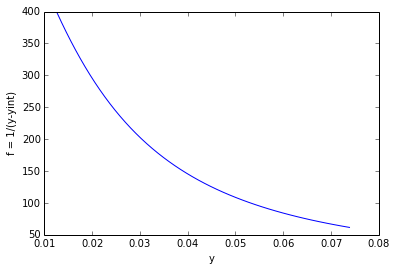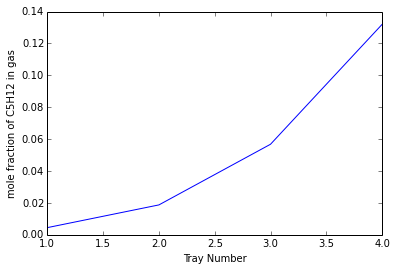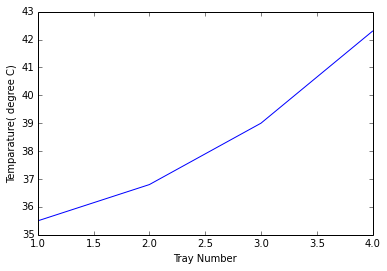# Chapter 5:Absorption and Stripping¶

### Example 5.1,Page number:287¶

In :
#Variable declaration

# Component 'A' is to be absorbed #
y_N1 = 0.018						# [mole fraction 'A' of in entering gas]
y_1 = 0.001						# [mole fractio of 'A'in leaving gas]
x_0 = 0.0001						# [mole fraction of 'A' in entering 							liquid]
m = 1.41						# [m = yi/xi]
n_1 = 2.115						# [molar liquid to gas ratio at bottom, 							L/V]
n_2 = 2.326						# [molar liquid to gas ratio at top, L/V]
E_MGE = 0.65

# Solution (a)

A_1 = n_1/m						# [absorption factor at bottom]
A_2 = n_2/m						# [absorption factor at top]
import math
from scipy.optimize import fsolve
A = math.sqrt(A_1*A_2)
# Using equation 5.3 to calculate number of ideal stages
N = (math.log(((y_N1-m*x_0)/(y_1-m*x_0))*(1-1/A) + 1/A))/math.log(A)	# [number of ideal 									stages]
print"Number of ideal trays is",round(N,3)
# Using equation 5.5
E_o = math.log(1+E_MGE*(1/A-1))/math.log(1/A)
# Therefore number of real trays will be
n = N/E_o

#Result

print"Number of real trays is",round(n,2)

print"Since it is not possible to specify a fractional number of trays, therefore number of real trays is",round(n)

# Solution (b)

#Calculation

N_o = E_o*n
# Putting N_o in equation 5.3 to calculate y_1
def f16(Z):
return(N_o-(math.log(((y_N1-m*x_0)/(Z-m*x_0))*(1-1/A) + 1/A))/math.log(A))
Z = fsolve(f16,0.001)
print"Mole fraction of A in leaving gas is",Z,"percent which satisfies the requirement that the gas exit concentration should not exceed 0.1 percent"

# For a tower diameter of 1.5 m, Table 4.3 recommends a plate spacing of 0.6 m
Z = n*0.6						# [Tower height, m]

#Result
print"The tower height will be",round(Z,1),"m"

Answer 5.1 (a)
Number of ideal trays is 4.647
Number of real trays is 7.79
Since it is not possible to specify a fractional number of trays, therefore number of real trays is 8.0

Mole fraction of A in leaving gas is 0.001 percent which satisfies the requirement that the gas exit concentration should not exceed 0.1 percent
The tower height will be 4.7 m


### Exmaple 5.3,Page number:295¶

In :
#Variable declaration
# For tower diameter, packed tower design program of Appendix D is run using # the data 		from Example 5.2 and packing parameters from Chapter 4.

# For a pressure drop of 300 Pa/m, the program converges to a tower diameter
Db = 0.641  						# [m]
# Results at the bottom of tower
fb= 0.733  						# [flooding]
ahb = 73.52 						# [m**-1]
Gmyb = 126  						# [mol/square m.s]
kyb = 3.417  						# [mol/square m.s]
klb = 9.74*10**-5  					# [m/s]

# From equation 2.6 and 2.11
# Fg = ky*(1-y),   Fl = kx*(1-x)
# Assume 1-y = 1-y1    1-x = 1-x1
# let t = 1-y1  u = 1-x1
# Therefore
t = 0.926
u = 0.676
Fgb = kyb*t  						# [mol/square m.s]
rowlb = 780  						# [kg/cubic m]
Mlb = 159.12  						# [gram/mole]
c = rowlb/Mlb  						# [kmle/cubic m]
Flb = klb*c*u  						# [mol/square m.s]
# From equ 5.19
Htgb = Gmyb/(Fgb*ahb)  					# [m]
import math
from scipy.optimize import fsolve
from numpy import *
from pylab import *
# Now, we consider the conditions at the top of the absorber
# For a pressure drop of 228 Pa/m, the program converges to a tower        # diameter
Dt = 0.641  						# [m]
# Results at the top of tower
ft = 0.668  						# [flooding]
aht = 63.31  						# [m**-1]
Gmyt = 118  						# [mol/square m.s]
kyt = 3.204  						# [mol/square m.s]
klt = 8.72*10**-5  					# [m/s]

rowlt = 765  						# [kg/cubic m]
Mlt = 192.7  						# [gram/mole]
cl = rowlt/Mlt  					# [kmole/cubic m]
Fgt = kyt*0.99  					# [mole/square m.s]
Flt = klb*cl*0.953  					# [mole/square m.s]
# From equ 5.19
Htgt = Gmyt/(Fgt*aht)  					# [m]
Htg_avg = (Htgb+Htgt)/2  				# [m]
Fg_avg = (Fgt+Fgb)/2  					# [mole/square m.s]
Fl_avg = (Flb+Flt)*1000/2  				# [mole/square m.s]

# The operating curve equation for this system in terms of mole fractions
# y =

# From Mathcad program figure 5.3
x1 = 0.324
x2 = 0.0476
n = 50
dx = (x1-x2)/n
me = 0.136
T = zeros((50,2))
y=zeros((50))
x=zeros((50))
yint=zeros((50))
fd=zeros((50))
for j in range(1,51):
x[j-1] = x2+j*dx
y[j-1] = (0.004+0.154*x[j-1])/(1.004-0.846*x[j-1])

def f12(yint):
return((1-yint)/(1-y[j-1]) - ((1-x[j-1])/(1-yint/me))**(Fl_avg/Fg_avg))
yint[j-1] = fsolve(f12,0.03)
fd[j-1] = 1/(y[j-1]-yint[j-1])
T[j-1] = y[j-1]

T[j-1] = fd[j-1]

#Result

a1=plot(T[:,0],T[:,1])

xlabel("y")
ylabel("f = 1/(y-yint)")

yo = y
yn = y
# From graph between f vs y
Ntg = 10.612
# Therefore
Z = Htg_avg*Ntg		  					# [m]

print"The total packed height is",round(Z),"m."
deltaPg = 300*Z 	 					# [Pa]
Em = 0.60 							 # [mechanical efficiency]
Qg = 1.0
Wg = (Qg*deltaPg)/Em  						# [Power required to force the 								gas through the tower, W]
L2 = 1.214  							# [kg/s]
g = 9.8  							# [m/square s]
Wl = L2*g*Z/Em  						# [Power required to pump the 								liquid to the top of the 								absorber, W]

print"The power required to force the gas through the tower is ",round(Wg),"W.\n\n"
print"The power required to pump the liquid to the top of the absorber is ",round(Wl),"W.\n\n"

show(a1)

The total packed height is 6.0 m.
The power required to force the gas through the tower is  2996.0 W.

The power required to pump the liquid to the top of the absorber is  119.0 W.### Example 5.4,Page number:299¶

In :
#Variable declaration
m = 0.57
D = 0.738  				# [tower diameter, m]
G = 180.0  				# [rate of gas entering the tower, kmole/h]
L = 151.5  				# [rate of liquid leaving the tower, kmole/h]
# Amount of ethanol absorbed
M = G*0.02*0.97  			# [kmole/h]

#Calculation

import math
# Inlet gas molar velocity
Gmy1 = G*4/(3600*math.pi*D**2)  	# [kmole/square m.s]
# Outlet gas velocity
Gmy2 = (G-M)*4/(3600*math.pi*D**2)  	# [kmole/square m.s]
# Average molar gas velocity
Gmy = (Gmy1+Gmy2)/2  			# [kmole/square m.s]

# Inlet liquid molar velocity
Gmx2 = L*4/(3600*math.pi*D**2)  	# [kmole/square m.s]
# Outlet liquid molar velocity
Gmx1 = (L+M)*4/(3600*math.pi*D**2)  	# [kmole/square m.s]

# Absorption factor at both ends of the column:
A1 = Gmx1/(m*Gmy1)
A2 = Gmx2/(m*Gmy2)
# Geometric average
A = math.sqrt(A1*A2)

y1 = 0.02
# For 97% removal of the ethanol
y2 = 0.03*0.02
# Since pure water is used
x2 = 0
# From equation 5.24
Ntog = math.log((y1-m*x2)/(y2-m*x2)*(1-1/A)+1/A)/(1-1/A)

# From example 4.4
# ky*ah = 0.191 kmole/cubic m.s
# kl*ah = 0.00733 s**-1
kyah = 0.191  				# [kmole/cubic m.s]
klah = 0.00733  			# [s**-1]
rowl = 986  				# [kg/cubic m]
Ml = 18.0  				# [gram/mole]
c = rowl/Ml  				# [kmole/cubic m]
kxah = klah*c  				# [kmole/cubic m.s]

# Overall volumetric mass transfer coefficient
Kyah = (kyah**-1 + m/kxah)**-1  	# [kmole/cubic m.s]

# From equation 5.22
Htog = Gmy/Kyah  				# [m]
# The packed height is given by equation 5.21,
Z = Htog*Ntog  					# [m]

#Result
print"The packed height of an ethanol absorber is",round(Z,2),"m.\n\n"

The packed height of an ethanol absorber is 5.66 m.



### Example 5.5,Page number:302¶

In :
#Variable declaration
# a = CH4 b = C5H12
Tempg = 27 							# [OC]
Tempo = 0 							# [base temp,OC]
Templ = 35 							# [OC]
xa = 0.75 							# [mole fraction of CH4 in gas]
xb = 0.25 							# [mole fraction of C5H12 in gas]
M_Paraffin = 200 						# [kg/kmol]
hb = 1.884 							# [kJ/kg K]

Ha = 35.59 							# [kJ/kmol K]
Hbv = 119.75 							# [kJ/kmol K]
Hbl = 117.53 							# [kJ/kmol K]
Lb = 27820 							# [kJ/kmol]
# M = [Temp (OC) m]
import math
import matplotlib
%matplotlib inline
from scipy.optimize import fsolve
from numpy import *
from pylab import *

#Calculation

M = matrix([[20,0.575],[25,0.69],[30,0.81],[35,0.95],[40,1.10],[43,1.25]])
# Basis: Unit time
GNpPlus1 = 1.0 							# [kmol]
yNpPlus1 = 0.25 						# [kmol]
HgNpPlus1 = ((1-yNpPlus1)*Ha*(Tempg-Tempo))+(yNpPlus1*(Hbv*(Tempg-Tempo)+Lb)) # [kJ/kmol]
L0 = 2.0 							# [kmol]
x0 = 0 								# [kmol]
HL0 = ((1-x0)*hb*M_Paraffin*(Templ-Tempo))+(x0*hb*(Templ-Tempo))	 # [kJ/kmol]
C5H12_absorbed = 0.98*xb 					# [kmol]
C5H12_remained = xb-C5H12_absorbed
G1 = xa+C5H12_remained 						# [kmol]
y1 = C5H12_remained/G1 						# [kmol]
LNp = L0+C5H12_absorbed 					# [kmol]
xNp = C5H12_absorbed/LNp 					# [kmol]
# Assume:
Temp1 = 35.6 							# [OC]
Hg1 = ((1-y1)*Ha*(Temp1-Tempo))+(y1*(Hbv*(Temp1-Tempo)+Lb)) 	# [kJ/kmol]
Qt = 0
def f30(HlNp):
return(((L0*HL0)+(GNpPlus1*HgNpPlus1))-((LNp*HlNp)+(G1*Hg1)+Qt))
HlNp = fsolve(f30,2)

def f31(TempNp):
return(HlNp-(((1-x0)*hb*M_Paraffin*(TempNp-Tempo))+(x0*hb*(TempNp-Tempo))))
TempNp = fsolve(f31,35.6)
# At Temp = TempNp:
mNp = 1.21
yNp = mNp*xNp 							# [kmol]
GNp = G1/(1-yNp) 						# [kmol]
HgNp = ((1-yNp)*Ha*(TempNp-Tempo))+(yNp*(Hbv*(TempNp-Tempo)+Lb)) # [kJ/kmol]
# From equation 5.28 with n = Np-1
def f32(LNpMinus1):
return(LNpMinus1+GNpPlus1-(LNp+GNp))
LNpMinus1 = fsolve(f32,2) 					# [kmol]

# From equation 5.29 with n = Np-1
def f33(xNpMinus1):
return(((LNpMinus1*xNpMinus1)+(GNpPlus1*yNpPlus1))-((LNp*xNp)+(GNp*yNp)))
xNpMinus1 = fsolve(f33,0) # [kmol]

# From equation 5.30 with n = Np-1
def f34(HlNpMinus1):
return(((LNpMinus1*HlNpMinus1)+(GNpPlus1*HgNpPlus1))-((LNp*HlNp)+(GNp*HgNp)))
HlNpMinus1 = fsolve(f34,0) # [kJ/kmol]
def f35(TempNpMinus1):
return(HlNpMinus1-(((1-xNpMinus1)*hb*M_Paraffin*(TempNpMinus1-Tempo))+(xNpMinus1*hb*(TempNpMinus1-Tempo))))
TempNpMinus1 = fsolve(f35,42) # [OC]

# The computation are continued upward through the tower in this manner until the gas 	composition falls atleast to 0.00662.
# Results = [Tray No.(n) Tn(OC) xn yn]
Results = matrix([[4.0,42.3,0.1091,0.1320],[3,39.0,0.0521,0.0568],[2,36.8,0.0184,0.01875],[1,35.5,0.00463, 0.00450]])
figure(1)
a1=plot(Results[:,0],Results[:,3])
xlabel("Tray Number")
ylabel("mole fraction of C5H12 in gas")
show(a1)
figure(2)
a2=plot(Results[:,0],Results[:,1])

xlabel("Tray Number")
ylabel("Temparature( degree C)")

show(a2)
# For the cquired y1
Np = 3.75

#result
print"The No. of trays will be",round(Np)The No. of trays will be 4.0